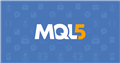# Define SL and TP mt5

hi guys,

I'm trying to write an EA. I have a problem with setting fixed sl and tp on my code. here's me code

```      double tp=300.00000;
double sl=100.00000;
double norm_tp=NormalizeDouble(tp, _Digits);
double norm_sl=NormalizeDouble(sl, _Digits);

I want to set TP at 300 pipets and SL at 100 pipet. I used NormalizeDouble function but it does'nt work.

any ideas?

yaser.amini67:

hi guys,

I'm trying to write an EA. I have a problem with setting fixed sl and tp on my code. here's me code

I want to set TP at 300 pipets and SL at 100 pipet. I used NormalizeDouble function but it does'nt work.

any ideas?

SL and TP must be PRICE values, not pips/points.

You are passing the wrong information.

For BUY orders TP, you need the get the current ASK Price, add the desired 300 points to the ASK Price respecting its decimal digits and normalize it.

The resulting Price is the TP price.

Example: you want TP to be 300 points. Considering EURUD as an example. Considering an Ask Price of 1.87654

300 must be converted to the correct decimal digits, which will be: 0.00300

Then add:  0.00300 + 1.87654 = 1.87954

Since TP parameter must be PRICE, in this example, TP will be 1.87954

Here is a code to get you started, which does the decimal adjust, sum, and normalization.

```int TP_Points = 300;
double norm_tp;

norm_tp = NormalizeDouble(Symbol.Ask() + (TP_Points * _Point), _Digits);

```

1. TP/SL are prices as @rrocchi says.

2. You buy at the Ask and sell at the Bid. Pending Buy Stop orders become market orders when hit and open at the Ask.

1. Your buy order's TP/SL (or Sell Stop's/Sell Limit's entry) are triggered when the Bid / OrderClosePrice reaches it. Using Ask±n, makes your SL shorter and your TP longer, by the spread. Don't you want the specified amount used in either direction?

MODE_SPREAD (Paul) - MQL4 programming forum - Page 3 #25

3. The charts show Bid prices only. Turn on the Ask line to see how big the spread is (Tools → Options (control+O) → charts → Show ask line.)
Most brokers with variable spreads widen considerably at end of day (5 PM ET) ± 30 minutes. My GBPJPY (OANDA) shows average spread = 26 points, but average maximum spread = 134 (your broker will be similar).

rrocchi:

SL and TP must be PRICE values, not pips/points.

You are passing the wrong information.

For BUY orders TP, you need the get the current ASK Price, add the desired 300 points to the ASK Price respecting its decimal digits and normalize it.

The resulting Price is the TP price.

Example: you want TP to be 300 points. Considering EURUD as an example. Considering an Ask Price of 1.87654

300 must be converted to the correct decimal digits, which will be: 0.00300

Then add:  0.00300 + 1.87654 = 1.87954

Since TP parameter must be PRICE, in this example, TP will be 1.87954

Here is a code to get you started, which does the decimal adjust, sum, and normalization.

Thank you for your reply but i'm getting the same error from before: failed instant sell [Invalid request]

I'm new with mql5 so maybe you can help me a little more. here is my code:

```//+------------------------------------------------------------------+
//|                                                       NewExp.mq5 |
//|                                                      Yaser Amini |
//|                                             https://www.mql5.com |
//+------------------------------------------------------------------+
#property version   "1.00"

int RSIH;
double RSI[];

int STOCH;
double Stoch[];
double DPer[];  // d%

int MACDH;
double MACD[];
double SIGNAL[];
double histogram_before;
double histogram_now;

int tp_points=300;
double norm_tp;

int sl_points=100;
double norm_sl;

//+------------------------------------------------------------------+
//| Expert initialization function                                   |
//+------------------------------------------------------------------+
int OnInit()
{
//---

RSIH=iRSI(_Symbol, _Period, 14, PRICE_CLOSE);
STOCH=iStochastic(_Symbol, _Period, 21, 5, 7, MODE_SMA, STO_CLOSECLOSE);
MACDH=iMACD(_Symbol, _Period, 12, 26, 9, PRICE_CLOSE);

ArraySetAsSeries(RSI, 1);
ArraySetAsSeries(Stoch, 1);
ArraySetAsSeries(DPer, 1);
ArraySetAsSeries(MACD, 1);
ArraySetAsSeries(SIGNAL, 1);

//---
return(INIT_SUCCEEDED);
}
//+------------------------------------------------------------------+
//| Expert deinitialization function                                 |
//+------------------------------------------------------------------+
void OnDeinit(const int reason)
{
//---

}
//+------------------------------------------------------------------+
//| Expert tick function                                             |
//+------------------------------------------------------------------+
void OnTick()
{

MqlTick Tick;
MqlRates Rates[];

CopyRates(_Symbol, _Period, 0, 2, Rates);
ArraySetAsSeries(Rates, 1);
SymbolInfoTick(_Symbol, Tick);

CopyBuffer(RSIH, 0, 0, 5, RSI);

CopyBuffer(STOCH, 0, 0, 5, Stoch);
CopyBuffer(STOCH, 1, 0, 5, DPer);

CopyBuffer(MACDH, 0, 0, 5, MACD);
CopyBuffer(MACDH, 1, 0, 5, SIGNAL);

histogram_before=MACD-SIGNAL;
histogram_now=MACD-SIGNAL;

bool SellPos=false;

if(PositionSelect(_Symbol)){

} else if(PositionGetInteger(POSITION_TYPE)==POSITION_TYPE_SELL){

SellPos=true;
}
}

return;
}

Print("Order Done!");

}
} else{
}
}

bool SellStoch=Stoch>DPer && Stoch<DPer;
bool SellRSI=RSI<=48;
bool SellMACD=MACD>SIGNAL && MACD<SIGNAL;

if(SellStoch && SellRSI && SellMACD){
if(SellPos){

return;
}

Print("Order Done!");
} else{
}
}

}
}
//+------------------------------------------------------------------+
```

As you can see i'm using ordersend() to my buy and sell executions. I'm not quite sure how to use sl and tp in this code. should I use sl and tp in both types of orders(buy and sell)?

yaser.amini67:

Thank you for your reply but i'm getting the same error from before: failed instant sell [Invalid request]

I'm new with mql5 so maybe you can help me a little more. here is my code:

As you can see i'm using ordersend() to my buy and sell executions. I'm not quite sure how to use sl and tp in this code. should I use sl and tp in both types of orders(buy and sell)?

Hmm.. your are doing it raw, via the hardest way to program. :)

I Suggest you use Ctrade to do everything. It facilitates a lot, and it does the hard job of validating and making sure everything is done correctly.

Since you are new on MQL5, start by learn how to use CTrade functions, because this is the best road ahead for programming EAs.

And its a lot more easy to program.• www.mql5.com

rrocchi:

Hmm.. your are doing it raw, via the hardest way to program. :)

I Suggest you use Ctrade to do everything. It facilitates a lot, and it does the hard job of validating and making sure everything is done correctly.

Since you are new on MQL5, start by learn how to use CTrade functions, because this is the best road ahead for programming EAs.

And its a lot more easy to program.